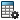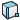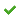Plotting Projection Angles for Rotating Parts

You can plot projection angles to specify the rotational orientation of a moving part.

1. Click Calculate(MotionManager toolbar).
2. In the PropertyManager, for Category, select Other Quantities.
3. For Subcategory select Projection Angles.
4. For Select result component, select one of the following:
• About X Axis to calculate rotation angle required to orient the Y-Z plane of a selected component with that of a reference frame.
• About Y Axis to calculate rotation angle required to orient the X-Z plane of a selected component with that of a reference frame.
• About Z Axis to calculate rotation angle required to orient the X-Y plane of a selected component with that of a reference frame.
5. Select features:
• Select a face of a part that rotates to calculate the rotation angle of the part orientation about the selected result component axis.
If you select a second face of a part that rotates in the same plane, the coordinates of the second part define the reference frame. Otherwise, global coordinates are used.
• Select a motion element such as a rotary motor, a torque, or a torsional spring. The reference frame is the global coordinates unless you select two components to define the motion element.
• Select a mate that permits rotation, such as a concentric mate to calculate the rotation angle along the selected axis of the orientation of the first part defining the mate with respect to that of the second part defining the mate.
6. Select the Plot Results options you require, and click.
The result appears in the MotionManager tree.
7. Click Calculate(MotionManager toolbar).